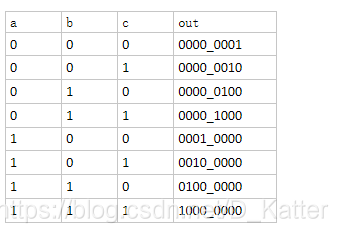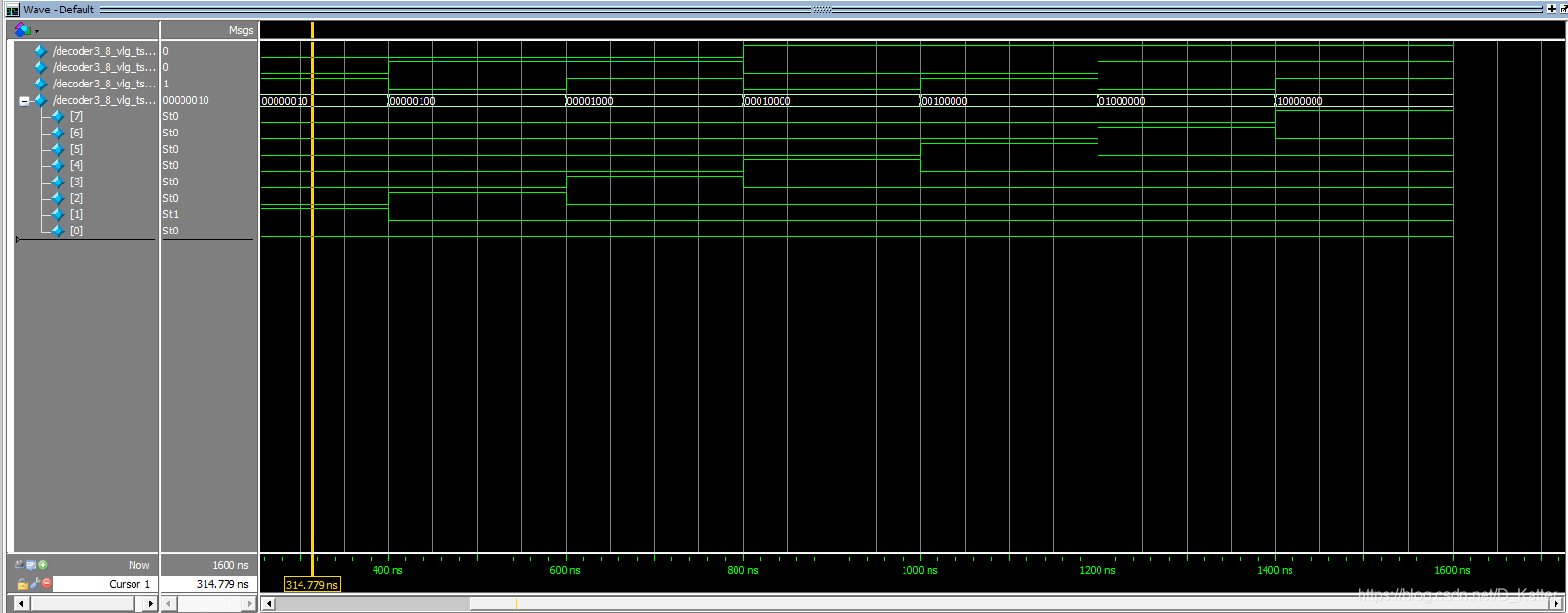FPGA基础知识（1）基本概念
FPGA学习（2）设计3-8译码器

07-01 21:14

02-13 21:54

02-13 21:52

01-10 22:39

01-08 18:51# FPGA学习（2）设计3-8译码器

## 2.真值表# 3.编写verilog代码

``````//声明
module decoder3_8(

a,b,c,
out

);
//说明
input a;
input b;
input c;
output [7:0]out;
reg [7:0] out;   //因为out在always块中使用，要定义成reg类型
//时序逻辑
always @(a,b,c)   //always@（*）

begin

case({a,b,c})

3'b000: out=8'b0000_0001;
3'b001: out=8'b0000_0010;
3'b010: out=8'b0000_0100;
3'b011: out=8'b0000_1000;
3'b100: out=8'b0001_0000;
3'b101: out=8'b0010_0000;
3'b110: out=8'b0100_0000;
3'b111: out=8'b1000_0000;
endcase
//可加default

end
endmodule
``````

## 4.编写仿真激励代码

``````//

`timescale 1 ns/ 1 ns   //设置精度
module decoder3_8_vlg_tst();
// constants
// general purpose registers

// test vector input registers
reg a;
reg b;
reg c;
// wires
wire [7:0]  out;

// assign statements (if any)
decoder3_8 i1 (
// port map - connection between master ports and signals/registers
.a(a),
.b(b),
.c(c),
.out(out)
);
initial
begin
// code that executes only once
// insert code here --> begin
a=0;b=0;c=0;
#200;
a=0;b=0;c=1;
#200;
a=0;b=1;c=0;
#200;
a=0;b=1;c=1;
#200;
a=1;b=0;c=0;
#200;
a=1;b=0;c=1;
#200;
a=1;b=1;c=0;
#200;
a=1;b=1;c=1;
#200;		//延时200ns
\$stop;

// --> end
\$display("Running testbench");
end

endmodule
``````

## 5.测试结果## 6.总结问题

verilog语言中的数据类型，在编写仿真文件的过程中，在case语句下，最开始编写的是：

`````` 3'b000: out=0000_0001;
3'b001: out=0000_0010;
3'b010: out=0000_0100;``````

（2）x和z的值

（3）负数“-”号必须写在最前面，比如-8‘b0000_0001（4）下划线下划线可以提高数字书写时，让程序更具有可读性，只能用在数字之间，也就是“_”的前面肯定是数字。比如写50MHz，可写成50_000_000Hz

0/200